Updating search results...

# 7 Results

View
Selected filters:
• Inside Mathematics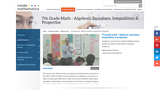Rating
0.0 stars

This lesson is about trying to get students to make connections between ideas about equations, inequalities, and expressions. The lesson is designed to give students opportunities to use mathematical vocabulary for a purpose to describe, discuss, and work with these symbol strings.The idea is for students to start gathering global information by looking at the whole number string rather than thinking only about individual procedures or steps. Hopefully students will begin to see the symbol strings as mathematical objects with their own unique set of attributes. (7th Grade Math)

Subject:
Algebra
Numbers and Operations
Material Type:
Activity/Lab
Lecture
Lesson Plan
Teaching/Learning Strategy
Provider:
Noyce Foundation
Provider Set:
Inside Mathematics
Author:
Disston, Jacob
10/10/2017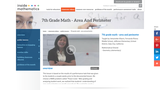Rating
0.0 stars

This lesson is based on the results of a performance task in which we realized that students' understanding of area and perimeter was mostly procedural. Therefore the purpose of this re-engagement lesson was to address student misconceptions and deepen student understanding of area and perimeter. The standards addressed in this lesson involve finding perimeter and area of various shapes, finding the perimeter when given a fixed area, and using a formula in a practical context. Challenges for our students included decoding the language in the problem and proving their thinking. (7th Grade Math)

Subject:
Mathematics
Material Type:
Activity/Lab
Lecture
Lesson Plan
Teaching/Learning Strategy
Provider:
Noyce Foundation
Provider Set:
Inside Mathematics
Author:
Villarin, Antoinette
10/10/2017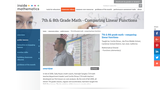Rating
0.0 stars

The foundation of this lesson is constructing, communicating, and evaluating student-generated tables while making comparisons between three different financial plans. Students are given three different DVD rental plans and asked to analyze each one to see if they could determine when the 3 different DVD plans cost the same amount of money, if ever. (7th/8th Grade Math)

Subject:
Algebra
Material Type:
Activity/Lab
Lecture
Lesson Plan
Teaching/Learning Strategy
Provider:
Noyce Foundation
Provider Set:
Inside Mathematics
Author:
Dimas, Cecilio
10/10/2017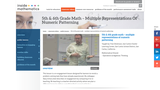Rating
0.0 stars

This lesson is a re-engagement lesson designed for learners to revisit a problem-solving task they have already experienced. Students will activate prior knowledge of graphical representations through the 'what's my rule' number talk; compare and contrast two different learners' interpretations of the growing pattern; use multiple representations to demonstrate how one of these learners would represent the numeric pattern; make connections between the different representations to more critically compare the two interpretations. (5th/6th Grade Math)

Subject:
Numbers and Operations
Material Type:
Activity/Lab
Lecture
Lesson Plan
Teaching/Learning Strategy
Provider:
Noyce Foundation
Provider Set:
Inside Mathematics
Author:
Dickinson, Fran
10/10/2017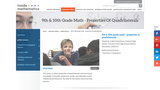Rating
0.0 stars

This lesson is about properties of quadrilaterals and learning to investigate, formulate, conjecture, justify, and ultimately prove mathematical theorems. Students will: Analyze characteristics and properties of two- and three-dimensional geometric shapes; develop mathematical arguments about geometric relationships; and apply appropriate techniques, tools, and formulas to determine measurements.Explore relationships among classes of two- and three-dimensional geometric objects, make and test conjectures about them, and solve problems involving them. Employ forms of mathematical reasoning and proof appropriate to the solution of the problem at hand, including deductive and inductive reasoning, making and testing conjectures, and using counter examples and indirect proof. Identify, formulate and confirm conjectures. Establish the validity of geometric conjectures using deduction, prove theorems, and critique arguments made by others. (9th/10th Grade Math)

Subject:
Algebra
Material Type:
Activity/Lab
Lecture
Lesson Plan
Teaching/Learning Strategy
Provider:
Noyce Foundation
Provider Set:
Inside Mathematics
Author:
Humphreys, Cathy
10/10/2017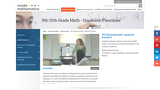Rating
0.0 stars

This lesson focuses on students making decisions about what tools to apply to solve different problems related to quadratic expressions and equations. It is also intended to build awareness of the form an answer will take in order to help students make sense of the kind of problem they are solving. (9th/10th/11th Grade Math)

Subject:
Algebra
Material Type:
Activity/Lab
Lecture
Lesson Plan
Teaching/Learning Strategy
Provider:
Noyce Foundation
Provider Set:
Inside Mathematics
Author:
Shreve, Barbara
10/10/2017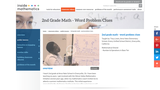Rating
0.0 stars

Students work to understand the language of word problems, using specific words as clues to the mathematical operations embedded in the problem. Teacher will use examples of student work to uncover misconceptions and errors and help support the students in developing the ability to critically evaluate their own strategies.Mathematics tasks include: Ability to read a problem in context and choose an operation to solve the problem; Ability to set up a number sentence that represents the problem; Accurately and efficiently solves two-digit addition and subtraction equations; Understands how to compose and decompose numbers; Can prove a sum or difference is correct using pictures, words, and/or symbols. (2nd Grade Math)

Subject:
Mathematics
Material Type:
Activity/Lab
Lecture
Lesson Plan
Teaching/Learning Strategy
Provider:
Noyce Foundation
Provider Set:
Inside Mathematics
Author:
Lewis, Tracy